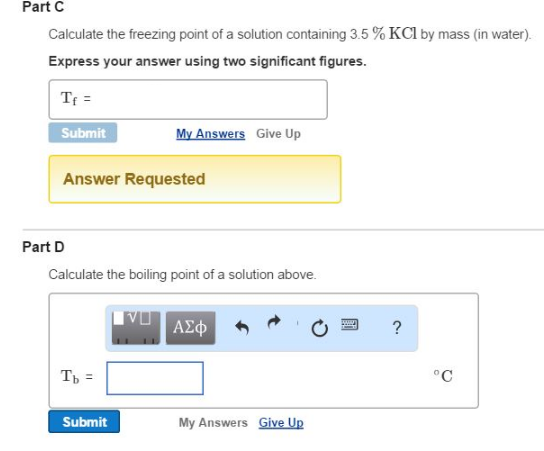# Problem: Part CCalculate the freezing point of a solution containing 3.5 % KCl by mass (in water). Express your answer using two significant figures. Part DCalculate the boiling point of a solution above.

###### FREE Expert Solution
96% (218 ratings)###### Problem Details

Part C

Calculate the freezing point of a solution containing 3.5 % KCl by mass (in water).

Part D

Calculate the boiling point of a solution above.